## Antenna Characteristics:

The following Antenna Characteristics isolated from surfaces which will alter or change their radiation patterns and efficiency.

### Current and Voltage Distribution:

When an RF signal voltage is applied at some point on an antenna, voltage and current will result at that point. Traveling waves are then initiated, and standing waves may be established, which means that voltage and current along the antenna are out of phase.

The radiation pattern depends chiefly on the antenna length measured in wavelengths, its power losses, and the terminations at its end (if any). In addition, the thickness of the antenna wire is of importance. For this discussion such Antenna Characteristics may be assumed to be lossless and made of wire whose diameter is infinitely small.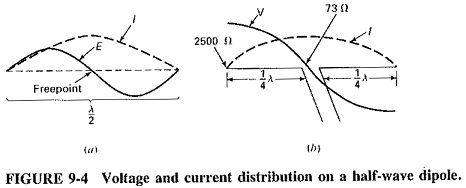Figure 9-4 shows the voltage and current distribution along a half-wave dipole. We can recognize the similarity to the distribution of voltage and current on a section of 1/4-λ transmission line open at the far end. These voltage and current characteristics are duplicated every λ/2 length, along the antenna (Figure 9-5).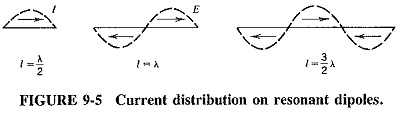By referring to Figure 9-4, it will become apparent that to connect a transmission line to this antenna configuration, we must observe the impedance at the connection points. The impedance varies along the length of the antenna, being highest where the current is lowest, and lowest where the current is highest (at the center). At the center of a half-wave antenna the impedance is approximately 73 Ω and increases to about 2500 Ω at either end.

In order to achieve maximum power transfer, this Antenna Characteristics must be connected to a 72 Ω transmission line. This method of connection, the transmission line to the antenna, is sometimes referred to as center or current fed.

### Resonant Antennas, Radiation Patterns, and Length Calculations:

Basic resonance theory has taught us that a high Q resonant circuit has a very narrow bandwidth. The same holds true for the resonant antenna. The narrow bandwidth establishes the useful limits for this type of radiator. This will be fully covered in Section 9-6.2.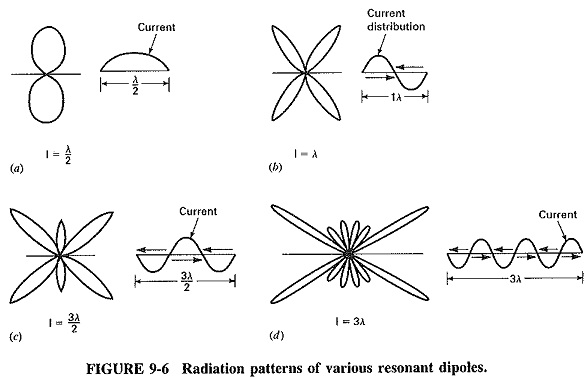The radiation pattern of a wire radiator in free space depends mainly on its length. Refer to Figure 9-6a for the standard figure eight pattern of a half wave. Figure 9-6b shows a full wave, Figure 9-6c a 1 1/2 wavelength, and Figure 9-6d three wavelengths.

The half-wave antenna has distributed capacitance and inductance and acts like a resonant circuit. The voltage and current will not be in phase. If an RF voltmeter is connected from the end of the antenna to ground, a large voltage will be measured. If the meter lead is moved toward the center, the voltage will diminish.

The length of the Antenna Characteristics can be calculated using Equation (9-3) (the velocity factor of wire is ≈95 percent compared to air, which is 1). ThenThis value is equal to one complete wavelength, and we can see that an antenna capable of transmitting, even at λ/2 (1111.5 ft) or λ/4 (555.75 ft), can be quite a structure. This size can become a problem at these lower frequencies.

Note that if we use the value 300 m/mHz (the speed of light), we can quickly calculate the physical length of a full-wave antenna in meters by recognizing that frequency and wavelength are inversely proportional.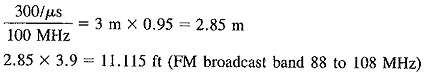This antenna, even at one full wavelength, is an easy structure to erect.

A half-wave dipole (Figure 9-6a) is like the elementary doublet (Figure 9-3), but somewhat flattened. The slight flattening of the pattern is due to the reinforcement at right angles to the dipole (called a figure eight pattern).

When the length of the antenna is one complete wavelength, the polarity of the current in one-half of the antenna is opposite to that on the other half (Figure 9-6b). As a result of these out-of-phase currents, the radiation at right angles from this antenna will be zero. The field radiated by one-half of the antenna alters the field radiated by the other half. A direction of maximum radiation still exists, but it is no longer at right angles to the antenna. For a full-wave dipole, maximum radiation will be at 54° to the antenna. This process has now generated extra lobes. There are four in this situation.

As the length of the dipole is increased to three half wavelengths, the current distribution is changed to that of Figure 9-6c. The radiation from one end of the antenna adds to that from the other, at right angles, but both are partially canceled by the radiation from the center, which carries a current of opposite polarity. There is radiation at right angles to the antenna, but it is not reinforced; therefore lobes in this direction are minor lobes. The direction of maximum radiation, or of major lobes, is closer to the direction or axis of the dipole itself, as shown in Figure 9-6d.

As we continue increasing the length, we increase the number of lobes, and the direction of the major lobes is brought closer or more aligned in the direction of the dipole. By looking closely at the patterns emerging, we can see that there are just as many radiation lobes on one side of the dipole as there are current lobes of both polarities. The 1 1/2 (3/2 λ) wavelength has three radiation lobes on each side, and a 3-λ antenna has six (Figure 9-6d).

### Nonresonant Antennas (Directional Antennas):

A nonresonant antenna, like a properly terminated transmission line, produces no standing waves. They are suppressed by the use of a correct termination resistor and no power is reflected, ensuring that only forwarding traveling waves will exist. In a correctly matched transmission line, all the transmitted power is dissipated in the terminating resistance. When an antenna is terminated as in Figure 9-7a, about two-thirds of the forward power is radiated; the remainder is dissipated in the Antenna Characteristics.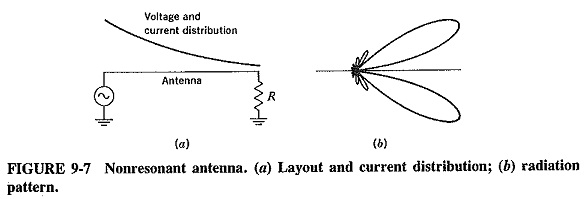As seen in Figure 9-7, the radiation patterns of the resonant antenna and a nonresonant one are similar except for one major difference. The nonresonant antenna is unidirectional. Standing waves exist on the resonant antenna, caused by the presence of both a reflected traveling wave and the forward traveling incident wave. The radiation pattern of the resonant antenna consists of two parts, as shown in Figure 9-8a and b, due to the forward and reflected waves. When these two processes are combined, the results are as shown in Figure 9-8c, and the familiar bidirectional pattern results.

Scroll to Top